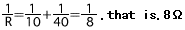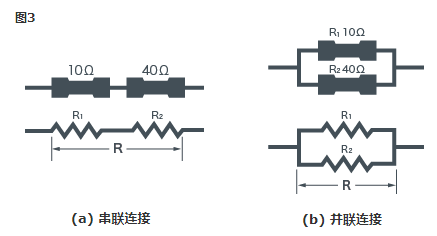# 什么是电阻器？ 基础知识

V=R・I…(1)

## 3. 那么，图1－(c)的电阻值多大？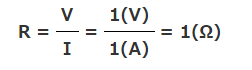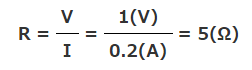V=R・I=5(Ω) × 1(A)=5(V)

VR=3(V)−2(V)=1(V)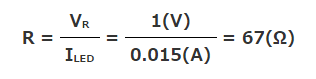P(W)=V(V)・I(A)

1(V)・1(A)=1(W)

## 5. 串联连接和并联连接

(a) 串联连接时合并电阻值…10Ω+40Ω=50Ω

(b) 并联连接时合并电阻值…